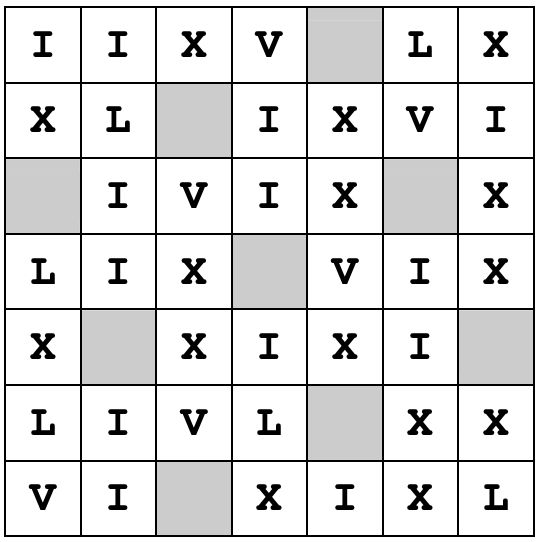시간 제한메모리 제한제출정답맞힌 사람정답 비율
1 초 128 MB153350.000%

## 문제

A square consists of cells organized in N rows and N columns. Each cell is either empty or contains a single Roman numeral (I, V, X, L, C, or D, representing numbers 1, 5, 10, 50, 100 and 500 respectively).

Look at the picture from third test example:We start at the central cell of the square (N will be odd and central cell will be empty in all test cases) and try to find the longest sequence of Roman numerals representing consecutive integers starting from 1 and separated by one empty cell. A sequence is obtained by "walking" through the square so that each step consists of moving to an adjacent cell left, right, up or down from the current cell. Each Roman numeral (including the last one) in a sequence must end with one (and only one) empty cell in a sequence. The output of a program should be (a decimal representation of) the largest number in the sequence.

Starting with the square above we can walk up-down-up-up-left-right-down-up-left to obtain the first tree numbers in a sequence IeIIeIIIe (where e denotes empty cell).

To obtain Roman representation of a number written in a decimal notation one may proceed as follows: Convert each decimal digit separately to its Roman equivalent taking its positional value into account, in descending order by their values. E.g. 726 = 700+20+6 → DCC XX VI = DCCXXVI. There are some special cases when letters representing Roman numeral of lower values are written before letters representing Roman numeral of higher values. Special cases here are numbers 4, 9, 40, 90 and 400 whose Roman numerals are obtained by writing lower numeral before higher: IV, IX, XL, XC and CD respectively. E.g. 499 = 400+90+9 = CD XC IX = CDXCIX (and not ID, as it might appearto be).

Write a program that will find the longest sequence of Roman numerals representing consecutive positive integer numbers starting from 1 and separated by one empty cell (as described above) and write (a decimal representation of) the largest number in the sequence.

## 입력

First line of input contains an odd integer N, 1 ≤ N ≤ 99.

Each of the next N lines contains N characters depicting the corresponding row of a square using characters 'I', 'V', 'X', 'L', 'C', 'D' and '.' (dot, denoting empty cell).

## 출력

First and only line of output should contain the decimal representation of the last number in the longest sequence of Roman numerals as described above.

## 예제 입력 1

3
I.I
I.V
I..


## 예제 출력 1

6


## 예제 입력 2

5
.IV.I
II.II
XV.VI
..I..
...II


## 예제 출력 2

11


## 예제 입력 3

7
IIXV.LX
XL.IXVI
.IVIX.X
LIX.VIX
X.XIXI.
LIVL.XX
VI.XIXL


## 예제 출력 3

51